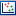## Principal component analysis

A principal component analysis is a mathematical analysis that identifies and quantifies the directions of variability in the data. For a set of samples, e.g. an experiment, this can be done by finding the eigenvectors and eigenvalues of the covariance matrix of the samples. The eigenvectors are orthogonal. The first principal component is the eigenvector with the largest eigenvalue, and specifies the direction with the largest variability. The second principal component is the eigenvector with the second largest eigenvalue, and specifies the direction with the second largest variability. Similarly for the third, etc. The data can be projected onto the space spanned by the eigenvectors. A plot of the data in the space spanned by the first and second principal component will show a simplified version of the data with variability in other directions than the two major directions of variability ignored.

To start the analysis:

Toolbox | Transcriptomics Analysis ()| Quality Control | Principal Component Analysis ()

Select a number of samples ( () or ()) or an experiment () and click Next.

This will display a dialog as shown in figure 27.81.Figure 27.81: Selcting which values the principal component analysis should be based on.

In this dialog, you select the values to be used for the principal component analysis (see Selecting transformed and normalized values for analysis).

Click Next if you wish to adjust how to handle the results. If not, click Finish.

Subsections